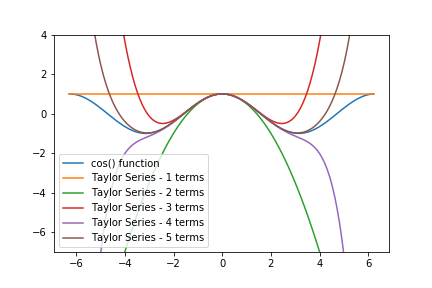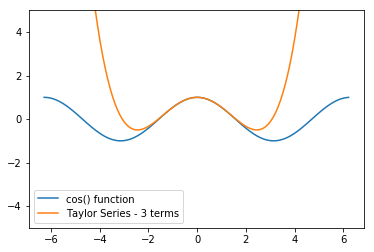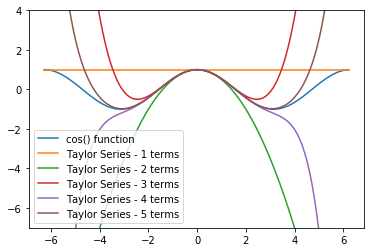In this post, we will review how to create a Taylor Series with Python and for loops. Then we will refactor the Taylor Series into functions and compare the output of our Taylor Series functions to functions from Python's Standard Library.

A Taylor Series is an infinite series of mathematical terms that when summed together approximate a mathematical function. A Taylor Series can be used to approximate $e^x$, and $cosine$.

An example of a Taylor Series that approximates $e^x$ is below.

$${e^x} \approx \sum\limits_{n = 0}^\infty {\frac{{{x^n}}}{{n!}}} \approx 1 + x + \frac{{{x^2}}}{{2!}} + \frac{{{x^3}}}{{3!}} + \frac{{{x^4}}}{{4!}} \ + \ ...$$

We can see that each term in the Taylor Series expansion is dependent on that term's place in the series. Below is a chart that shows each term of the Taylor Series in a row. The columns of the table represent the term index, the mathematical term and, how to code that term in Python. Note that the factorial() function is part of the math module in Python's Standard Library.

Term Index Mathematical Term Term coded in Python
0 $x^0/0!$ x**0/math.factorial(0)
1 $x^1/1!$ x**1/math.factorial(1)
2 $x^2/2!$ x**2/math.factorial(2)
3 $x^3/3!$ x**3/math.factorial(3)
4 $x^4/4!$ x**4/math.factorial(4)

## Code the Taylor Series by writing out each term individually¶

We can combine these terms in a line of Python code to estimate $e^2$. The code below calculates the sum of the first five terms of the Taylor Series expansion of $e^x$, where $x=2$. Note the math module needs to be imported before math.factorial() can be used.

In :
import math

x = 2
e_to_2 = x**0/math.factorial(0) + x**1/math.factorial(1) + x**2/math.factorial(2) + x**3/math.factorial(3) + x**4/math.factorial(4)
print(e_to_2)

7.0


Our Taylor Series approximation of $e^2$ was calculated as 7.0. Let's compare our Taylor Series approximation to Python's math.exp() function. Python's math.exp() function raises $e$ to any power. In our case, we want to use math.exp(2) because we want to calculate $e^2$.

In :
print(math.exp(2))

7.38905609893065


Our Taylor Series approximation 7.0 is not that far off the calculated value 7.389056... using Python's exp() function.

## Use a for loop to calculate a Taylor Series¶

If we want to get closer to the value of $e^x$, we need to add more terms to our Taylor Series. The problem is coding each individual term is time-consuming and repetitive. Instead of coding each term individually, we can use a for loop. A for loop is a repetition structure in Python that runs a section of code a specified number of times. The syntax for coding a for loop in Python using the range() function is below:

for <var> in range(<num>):
<code>

Where <var> is any valid Python variable name and <num> is an integer to determines how many times the <code> in the loop runs.

We can recreate our approximation of $e^2$ with 5 terms using a for loop. Note we need to set the variable e_to_2 to 0 before the loop starts. The mathematical operator += in the line e_to_2 += x**i/math.factorial(i) is equivalent to e_to_2 = e_to_2 + x**i/math.factorial(i).

In :
import math

x = 2
e_to_2 = 0
for i in range(5):
e_to_2 += x**i/math.factorial(i)

print(e_to_2)

7.0


The result 7.0 is the same as the result we calculated when we wrote out each term of the Taylor Series individually.

An advantage of using a for loop is that we can easily increase the number of terms. If we increase the number of times the for loop runs, we increase the number of terms in the Taylor Series expansion. Let's try 10 terms. Note how the line for i in range(10): now includes 10 passed to the range() function.

In :
import math

x = 2
e_to_2 = 0
for i in range(10):
e_to_2 += x**i/math.factorial(i)

print(e_to_2)

7.3887125220458545


The result is 7.38871.... Let's see how close that is to $e^2$ calculated with Python's exp() function.

In :
print(math.exp(2))

7.38905609893065


The result is 7.38905.... We get closer to the value of $e^2$ when 10 terms are used in the Taylor Series compared to when 5 terms were used in the Taylor Series.

## Refactor the for loop into a function¶

Next, let's refactor the code above that contained a for loop (which calculated $e^2$) into a function that can compute $e$ raised to any power estimated with any number of terms in the Taylor Series. The general syntax to define a function in Python is below.

def <func name>(<arg 1>, <arg 2>, ...):
<code>
return <output>

Where def is the Python keyword that defines a function, <func name> is a valid Python variable name, and <arg 1>, <arg 2> are the input arguments passed to the function. <code> that runs when the function is called must be indented (the standard indentation is 4 spaces). The keyword return denotes the <output> of the function.

Let's code our for loop that approximates $e^2$ into a function.

In :
import math

def func_e_to_2(n):
x = 2
e_to_2 = 0
for i in range(n):
e_to_2 += x**i/math.factorial(i)

return e_to_2


If we call our function func_e_to_2() with the input argument 10, the result is the same as when we ran the for loop 10 times.

In :
out = func_e_to_2(10)
print(out)

7.3887125220458545


The output of our func_e_to_2() function is 7.38871....

We can make our function more general by setting x (the number that $e$ gets raised to) as an input argument. Note how now there are two input arguments in the function definition (x, n). x is the number $e$ is raised to, and n is the number of terms in the Taylor Series (which is the number of times the for loop runs on the inside of the function definition).

In :
import math

def func_e(x, n):
e_approx = 0
for i in range(n):
e_approx += x**i/math.factorial(i)

return e_approx


Let's calculate $e^2$ using 10 terms with our new func_e() function.

In :
out = func_e(2,10)
print(out)

7.3887125220458545


The result is 7.38871..., the same result as before.

An advantage to writing our Taylor Series expansion in a function is that now the Taylor Series approximation calculation is reusable and can be called in one line of code. For instance, we can estimate the value of $e^5$ with 10 terms, by calling our func_e() function with the input arguments (5,10).

In :
out = func_e(5,10)
print(out)

143.68945656966488


The result is 143.68945.... Let's see how close this value is to Python's exp() function when we make the same calculation $e^5$.

In :
out = math.exp(5)
print(out)

148.4131591025766


The result is 148.41315.... The Taylor Series approximation calculated by our func_e() function is pretty close to the value calculated by Python's exp() function.

## Use a for loop to calculate the difference between the Taylor Series expansion and Python's exp() function¶

Now let's use a for loop to calculate the difference between the Taylor Series expansion as calculated by our func_e() function compared to Python's exp() function. We'll calculate the difference between the two functions when we use between 1 and 10 terms in the Taylor Series expansion.

The code below uses f-strings, which is a Python syntax for inserting the value of a variable in a string. The general syntax for an f-string in Python is below.

f'string statment {<var>}'

Where f denotes the beginning of an f-string, the f-string is surrounded by quotes ' ', and the variable <var> is enclosed in curly braces { }. The value of <var> will be printed out without the curly braces.

In :
import math

x = 5
for i in range(1,11):
e_approx = func_e(x,i)
e_exp = math.exp(x)
e_error = abs(e_approx - e_exp)
print(f'{i} terms: Taylor Series approx= {e_approx}, exp calc= {e_exp}, error = {e_error}')

1 terms: Taylor Series approx= 1.0, exp calc= 148.4131591025766, error = 147.4131591025766
2 terms: Taylor Series approx= 6.0, exp calc= 148.4131591025766, error = 142.4131591025766
3 terms: Taylor Series approx= 18.5, exp calc= 148.4131591025766, error = 129.9131591025766
4 terms: Taylor Series approx= 39.33333333333333, exp calc= 148.4131591025766, error = 109.07982576924327
5 terms: Taylor Series approx= 65.375, exp calc= 148.4131591025766, error = 83.0381591025766
6 terms: Taylor Series approx= 91.41666666666667, exp calc= 148.4131591025766, error = 56.99649243590993
7 terms: Taylor Series approx= 113.11805555555556, exp calc= 148.4131591025766, error = 35.29510354702104
8 terms: Taylor Series approx= 128.61904761904762, exp calc= 148.4131591025766, error = 19.79411148352898
9 terms: Taylor Series approx= 138.30716765873015, exp calc= 148.4131591025766, error = 10.105991443846449
10 terms: Taylor Series approx= 143.68945656966488, exp calc= 148.4131591025766, error = 4.723702532911716


Note how the error decreases as we add terms to the Taylor Series. When the Taylor Series only has 1 term, the error is 147.41.... When 10 terms are used in the Taylor Series, the error goes down to 4.7237....

## Use a break statement to exit a for loop early.¶

How many terms would it take to produce an error of less than 1? We can use a break statement to drop out of the for loop when the error is less than 1. The code below calculates how many terms are needed in the Taylor Series, when $e^5$ is calculated, to keep the error less than 1.

In :
import math

x = 5
for i in range(1,20):
e_approx = func_e(x,i)
e_exp = math.exp(x)
e_error = abs(e_approx - e_exp)
if e_error < 1:
break

print(f'{i} terms: Taylor Series approx= {e_approx}, exp calc= {e_exp}, error = {e_error}')

12 terms: Taylor Series approx= 147.60384850489015, exp calc= 148.4131591025766, error = 0.8093105976864479


The output shows that it takes 12 terms in the Taylor Series to drop the error below 1.

## Create a function to estimate the value of $cos(x)$ using a Taylor Series¶

Next, let's calculate the value of the cosine function using a Taylor Series. The Taylor Series expansion for $\cos(x)$ is below.

$$\cos \left( x \right) \approx \sum\limits_{n = 0}^\infty {{{\left( { - 1} \right)}^n}\frac{{{x^{2n}}}}{{\left( {2n} \right)!}}} \approx 1 - \frac{{{x^2}}}{{2!}} + \frac{{{x^4}}}{{4!}} - \frac{{{x^6}}}{{6!}} + \frac{{{x^8}}}{{8!}} - \frac{{{x^{10}}}}{{10!}} \ + \ ...$$

We can code this formula into a function that contains a for loop. Note the variable x is the value we are trying to find the cosine of, the variable n is the number of terms in the Taylor Series, and the variable i is the loop index which is also the Taylor Series term number. We are using a separate variable for the coefficient coef which is equal to $(-1)^i$, the numerator num which is equal to $x^{2i}$ and the denominator denom which is equal to $(2i!)$. Breaking the Taylor Series formula into three parts can cut down on coding errors.

In :
import math

def func_cos(x, n):
cos_approx = 0
for i in range(n):
coef = (-1)**i
num = x**(2*i)
denom = math.factorial(2*i)
cos_approx += ( coef ) * ( (num)/(denom) )

return cos_approx


Let's use our func_cos() function to estimate the cosine of 45 degrees. Note that func_cos() function computes the cosine of an angle in radians. If we want to calculate the cosine of 45 degrees using our function, we first have to convert 45 degrees into radians. Luckily, Python's math module has a function called radians() that makes the angle conversion early.

In :
angle_rad = (math.radians(45))
print(out)

0.7071068056832942


Using our func_cos() function and 5 terms in the Taylor Series approximation, we estimate the cosine of 45 degrees is 0.707106805.... Let's check our func_cos() function compared to Python's cos() function from the math module.

In :
out = math.cos(angle_rad)
print(out)

0.7071067811865476


Using Python's cos() function, the cosine of 45 degrees returns 0.707106781... This value is very close to the approximation calculated using our func_cos() function.

## Build a plot to compare the Taylor Series approximation to Python's cos() function¶

In the last part of this post, we are going to build a plot that shows how the Taylor Series approximation calculated by our func_cos() function compares to Python's cos() function.

The idea is to make a plot that has one line for Python's cos() function and lines for the Taylor Series approximation based on different numbers of terms.

For instance, if we use 3 terms in the Taylor Series approximation, our plot has two lines. One line for Python's cos() function and one line for our func_cos() function with three terms in the Taylor series approximation. We'll calculate the cosine using both functions for angles between $-2\pi$ radians and $2\pi$ radians.

In :
import math
import numpy as np
import matplotlib.pyplot as plt
# if using a Jupyter notebook, include:
%matplotlib inline

angles = np.arange(-2*np.pi,2*np.pi,0.1)
p_cos = np.cos(angles)
t_cos = [func_cos(angle,3) for angle in angles]

fig, ax = plt.subplots()
ax.plot(angles,p_cos)
ax.plot(angles,t_cos)
ax.set_ylim([-5,5])
ax.legend(['cos() function','Taylor Series - 3 terms'])

plt.show()We can use a for loop to see how much better adding additional terms to our Taylor Series approximation compares to Python's cos() function.

In :
import math
import numpy as np
import matplotlib.pyplot as plt
# if using a Jupyter notebook, include:
%matplotlib inline

angles = np.arange(-2*np.pi,2*np.pi,0.1)
p_cos = np.cos(angles)

fig, ax = plt.subplots()
ax.plot(angles,p_cos)

# add lines for between 1 and 6 terms in the Taylor Series
for i in range(1,6):
t_cos = [func_cos(angle,i) for angle in angles]
ax.plot(angles,t_cos)

ax.set_ylim([-7,4])

# set up legend
legend_lst = ['cos() function']
for i in range(1,6):
legend_lst.append(f'Taylor Series - {i} terms')
ax.legend(legend_lst, loc=3)

plt.show()We see the Taylor Series with 5 terms (the brown line) comes closest to approximating Python's cos() function. The Taylor Series with 5 terms is a good approximation of the cosine of angles between about $-\pi$ and $\pi$ radians. The Taylor Series with 5 terms is a worse approximation for angles less than $-\pi$ or greater than $\pi$. As the angle gets further away from zero radians, the estimate of the cosine using a Taylor Series gets worse and worse.

## Summary¶

In this post, we reviewed how to construct the Taylor Series of $e^x$ and $\cos(x)$ in Python. First, we coded each term of the Taylor series individually. Next, we used a for loop to calculate $n$ terms in the Taylor Series expansion. We then refactored the code with the for loop into a function and parameterized our function so that we could calculate $e$ raised to any number calculated out to any number of terms. At the end of the post, we coded the Taylor Series of $\cos(x)$ into a Python function. Finally, we used our Taylor Series cosine function to build a plot with Matplotlib that shows how the Taylor Series approximation compares to Python's cos() function for angles between $-2\pi$ and $2\pi$ radians.